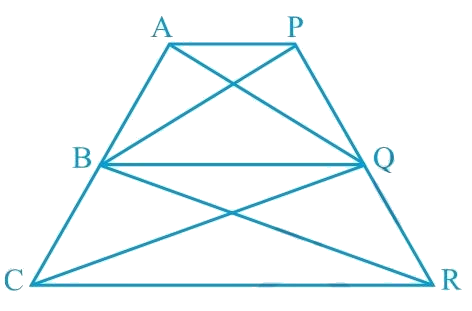# Ex.9.3 Q14 Areas of Parallelograms and Triangles Solution - NCERT Maths Class 9

## Question

In the given figure, $$AP \;|| \;BQ \;|| \;CR$$. Prove that $$ar (AQC) = ar (PBR)$$.

Video Solution
Areas Of Parallelograms And Triangles
Ex 9.3 | Question 14

## Text Solution

What is known?

$$AP || BQ || CR.$$

What is unknown?

How we can prove that $$ar (AQC) = ar (PBR).$$

Reasoning:

We can use theorem for triangles $$ABQ$$ and $$PBQ$$, if two triangles are on same base and between same pair of parallel lines then both will have equal area. Similarly for triangles we can use for triangles $$BCQ$$ and $$BRQ$$. Now by adding both the results, we will get the required result.

Steps:Since \begin{align}\rm \Delta ABQ\, \rm and \,\Delta PBQ\end{align} lie on the same base $$BQ$$ and are between the same parallels $$AP$$ and $$BQ$$,

According to Theorem 9.2 : Two triangles on the same base (or equal bases) and between the same parallels are equal in area.

\begin{align}\therefore \text {ar}(\Delta {ABQ})\!=\!\text {ar}(\triangle {PBQ})\dots\text (1)\end{align}

\begin{align} (\Delta BCQ)\, and \,(\Delta BRQ)\end{align} lie on the same base  and are between the same parallels  and .

\begin{align}\therefore \text {ar}(\Delta B C Q)\!=\!\text {ar}(\Delta B R Q) \ldots \text (2)\end{align}

On adding Equations ($$1$$) and ($$2$$), we obtain

$\left[ \begin{array}{l}{{ar}}(\Delta {{ABQ}}) + \\{{ar}}(\Delta {{BCQ}})\end{array} \right] = \left[ \begin{array}{l}{{ar}}(\Delta {{PBQ}})\!+\!\\{{ar}}(\Delta {{BRQ}})\end{array} \right]$

\begin{align} {\therefore \text {ar}(\Delta {AQC})}&\!=\!{ {ar}(\Delta {PBR})}\end{align}

Video Solution
Areas Of Parallelograms And Triangles
Ex 9.3 | Question 14
Learn from the best math teachers and top your exams

• Live one on one classroom and doubt clearing
• Practice worksheets in and after class for conceptual clarity
• Personalized curriculum to keep up with school